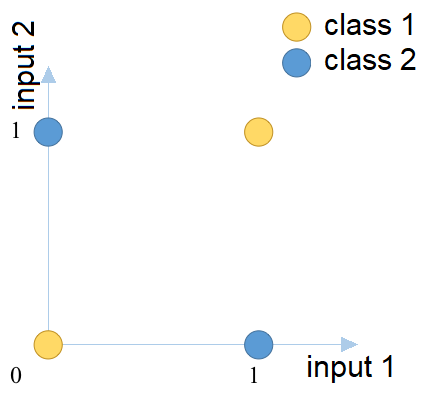# Context¶

The XOR problem is a known classification problem, where a two dimensional input space is mapped to a single variable as shown in the table and figure below:

Input 1 Input 2 Output Class
False False A
False True B
True False B
True True AThe XOR classification problem, represented on a graph.

A classification problem like this, where the different classes are no longer linearly separable cannot be solved with e.g. a minimum distance or a maximum likelihood classifier.

Linearly inseparable classification problems are in practice the rule, rather than the exception. They can be circumvented by splitting up clusters in the input space into multiple sub-clusters before classification, and then merge them again after classification (see table below). However, this is only possible if we have knowledge on the division of the classes in the input space.

Input 1 Input 2 Output Class
False False A
False True B
True False B
True True C

Artificial Neural Networks are particularly suited to solve this type of linearly inseparable classification problems. The division between the different classes are determined in an iterative process:

1. Training data is presented to the network.
2. The network guesses the output based on internal parameters (weights).
3. This output is compared to the training data and the network error is calculated.
4. The derivative of the network error to each individual weight is calculated.
5. The weights are adjusted in the opposite direction of that derivative.
6. Repeat a fixed number of times, or until the network error falls below a given threshold.